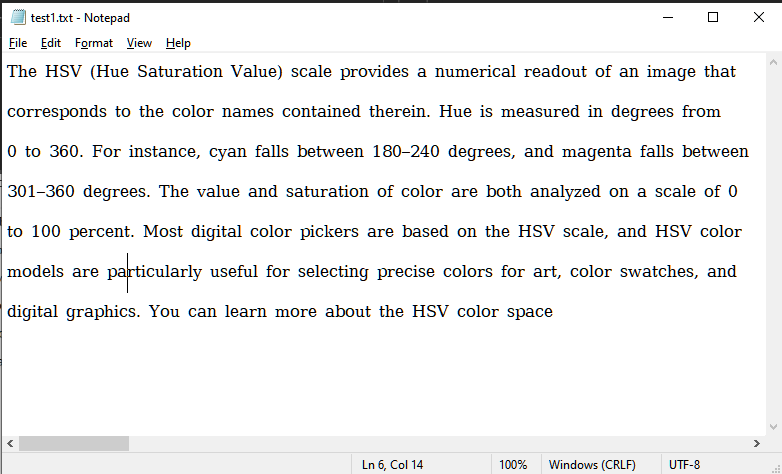GeeksforGeeks App
Open AppBrowser
Continue

# Find line number of a specific string or substring or word from a .txt file in Python

Finding the line number of a specific string and its substring is a common operation performed by text editors or any application with some level of text processing capabilities.

In this article, you will learn how to find line number of a specific string or substring or word from a .txt (plain text) file using Python

The problem in hand could be stretched to include different methods. i.e. The line number of the first occurrence of the substring/string could be found, or the line numbers at which the string/substring exists could be found. This article will be going over the latter one.

For demonstration the following text file would be used:### Finding single word in a text file

In this part, we will search for a single word in the text file. Let’s see the code here.

## Python3

 `# Name of the file in which the string is to be searched``filename ``=` `r``"test1.txt"` `# The string that is to be searched``key ``=` `"the"` `# Opening the file and storing its data into the variable lines``with ``open``(filename) as ``file``:``    ``lines ``=` `file``.readlines()` `# Going over each line of the file``for` `number, line ``in` `enumerate``(lines, ``1``): ``    ` `    ``# Condition true if the key exists in the line``    ``# If true then display the line number``    ``if` `key ``in` `line: ``        ``print``(f``'{key} is at line {number}'``) `

Output:

```"the" is at line 2
"the" is at line 5
"the" is at line 7```

Explanation:

Firstly the variables filename and key were initialized. The variable filename stores the path (absolute or relative) of the text file. The variable key stores the string that is to be searched. Afterward, the file at the path specified by the filename variable is opened for the read operation. All the lines within the files are read and stored in the variable lines. A for-loop is ran where this variable is passed through the enumerate function to get each line along with its number as the condition. Therefore, the loop would execute until all the elements of the lines list are exhausted. Then a condition is placed inside the loop, which searches for the key within the line using the in operator. If the presence is found, the line at which the key is found is printed, and this process repeats until all the lines within the file are checked.

### Finding different words in a text file

In this part, we will search for a different words in the text file. Let’s see the code here.

## Python3

 `# Name of the file in which the string is to be searched``filename ``=` `r``"test1.txt"` `# The list containing the strings that are to be searched``key ``=` `[``"the"``, ``"and"``, ``"or"``, ``"to"``]` `# Opening the file and storing its data into the variable lines``with ``open``(filename) as ``file``:``    ``lines ``=` `file``.readlines()` `# Going over each element of the list``for` `x ``in` `key:` `    ``# Same as the one for finding only one key``    ``for` `number, line ``in` `enumerate``(lines, ``1``):     ``        ``if` `x ``in` `line: ``            ``print``(f``'{x} is at line {number}'``) `

Output:

```the is at line 2
the is at line 5
the is at line 7
and is at line 3
and is at line 4
and is at line 5
and is at line 6
or is at line 2
or is at line 3
or is at line 4
or is at line 5
or is at line 6
or is at line 7
to is at line 2
to is at line 3
to is at line 5```

Explanation:

The working logic of the code is safe as the aforementioned one. The only difference being that we are supplying more than 1 key to be searched into the file in a loop. Therefore, in this way we can find many different substrings within the file, at the same time.

### Finding different substrings in a text file

In this part, we will search for  substring in the text file. Let’s see the code here.

## Python3

 `def` `search(key, ``file``):``    ``# Opening the file and storing its data into the variable lines``    ``with ``open``(filename) as ``file``:``        ``lines ``=` `file``.readlines()``    ``for` `number, line ``in` `enumerate``(lines, ``1``):``        ``# Going over each key``        ``if` `key ``in` `line:``            ``print``(f``'{key} is at line {number}'``)`  `# Name of the file in which the string is to be searched``filename ``=` `r``"test1.txt"``key ``=` `"in hand"``string_list ``=` `list``(key.split())``for` `item ``in` `string_list:``    ``search(item, filename)`

Output:

```in is at line 1
in is at line 3
hand is at line 3```

My Personal Notes arrow_drop_up